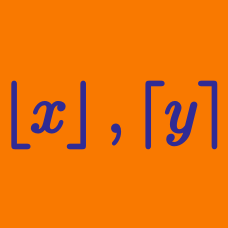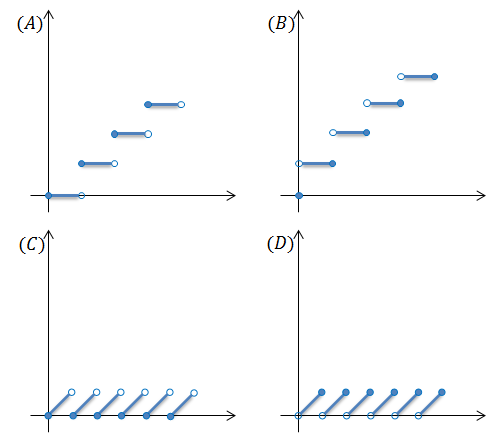Algebra

# Floor and Ceiling Functions - Problem Solving

How many $0$'s are on the end of $180!?$

Note: $180!$ is the factorial of $180,$ which means $180\times179\times178\times\cdots\times2\times1.$

The positive root of $20x^2=\lceil x \rceil$ is $A.$ Find the value of $\frac{1}{A^2}.$

Evaluate $\sum_{i=1}^{44}\left\lfloor\frac{16i}{45}\right\rfloor.$

Evaluate

$\sum_{i=1}^{ 128 } \lfloor \log_2 i \rfloor .$Which of the figures above correctly illustrates the graph of $y=\{x\}?$

Note: $\{x\}$ denotes the fractional part function.

×